Rapid Revision For School Students

# KS2 Maths Quizzes

##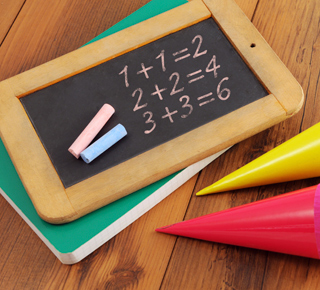Learning KS2 Maths: Numbers and Solutions

(Fun KS2 Maths revision quizzes to teach students in Year 3, Year 4, Year 5 and Year 6)

KS2 means stepping the game up in mathematics.

You’ve already said a cheery hello to addition, number lines and multiplication. Now it’s time to get stuck in with fractions, shapes, perimeters and other mathematically-spectacular topics. KS2 students can refine their skills to achieve the much-desired Mathematical Whizz Kid status.

We think anyone who makes it through all our KS2 quizzes on maths deserves a little ‘hip hip hooray’ (we would pull party poppers too if we could!).

We won’t lie to you - it’s not all ice cream and sprinkles down there in the quiz section. Some of this stuff gets tricky. Really tricky. Sometimes you’ll be scratching your head like the ponderer you are, trying to digest all the new information and wondering if you’ll ever be able to figure out percentages or ratios. And then it will happen. You’ll shout ‘Eureka!’, you’ll jump from your chair so high you’ll almost hit the roof and everything in your brain will slot nicely into place. That’s the magic in maths and we’ve designed our quizzes to try to make that happen as often as possible.

We’ll provide helpful explanations after each incorrect answer to let you know where you went wrong and (most importantly) teach you how to get the right answer next time.

Maths in the classroom is a little pernickety. (Do you like that word, pernickety? We love it. What a great word.) In a single lesson, maths can go from simple to mind-bogglingly complicated. That’s where we step in. If you come out of maths classes a little bit confused, or not entirely sure if you were doing it right, we’ll be here to act as a revision tool to go over what you’ve covered. We include the key nuggets from the National Curriculum, so we know what to teach you!

We’re training the next generation of Mathematical Whizz Kids.

Let’s see who’s the smartest cookie in the box.

To find out more about this curriculum, our Key Stage 2 (KS2) article will keep you informed.

Did you know...
...we do test papers too? Click here to view our KS2 Maths Quizzes test papersQuiz Title Options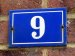Addition and Subtraction (Year 3)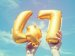Addition and Subtraction (Year 4)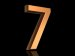Addition and Subtraction (Year 5)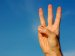Addition and Subtraction (Year 6)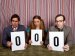Compare and Order Numbers (Year 4)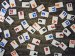Compare and Order Numbers (Year 5)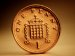Decimal Numbers (Year 4)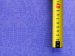Decimal Numbers (Year 5)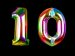Decimal Numbers (Year 6)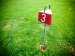Fractions (Year 3)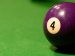Fractions (Year 4)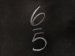Fractions (Year 5)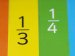Fractions (Year 6)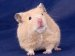Handling Data (Year 4)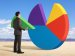Handling Data (Year 6)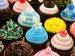Measures (Year 3)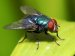Measures (Year 4)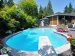Measures (Year 5)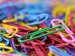Measures (Year 6)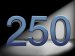Multiples (Year 3)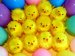Multiples (Year 4)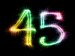Multiplication and Division (Year 3)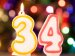Multiplication and Division (Year 4)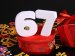Multiplication and Division (Year 5)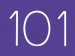Multiplication and Division (Year 6)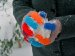Negative Numbers (Year 5)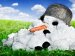Negative Numbers (Year 6)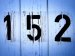Number Sequences (Year 3)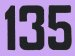Number Sequences (Year 4)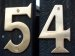Number Sequences (Year 5)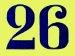Number Sequences (Year 6)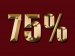Percentages (Year 5)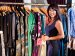Percentages (Year 6)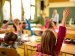Perimeter and Area (Year 4)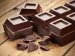Perimeter and Area (Year 5)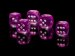Perimeter and Area (Year 6)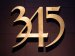Place Value (Year 3)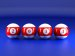Place Value (Year 4)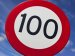Place Value (Year 5)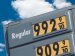Place Value (Year 6)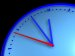Position (Year 3)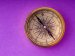Position (Year 4)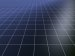Position (Year 5)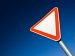Position (Year 6)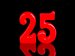Properties of Numbers (Year 5)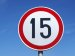Properties of Numbers (Year 6)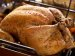Ratio (Year 5)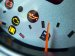Ratio (Year 6)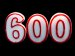Rounding Numbers (Year 3/4)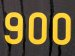Rounding Numbers (Year 5/6)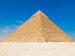Shapes (Year 3)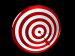Shapes (Year 4)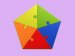Shapes (Year 5)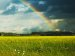Shapes (Year 6)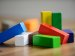Solving Problems (Year 3)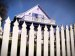Solving Problems (Year 4)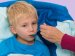Solving Problems (Year 5)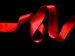Solving Problems (Year 6)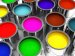Solving Problems - Money (Year 3)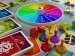Solving Problems - Money (Year 4)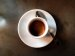Solving Problems - Money (Year 5)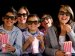Solving Problems - Money (Year 6)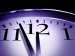Time (Year 3)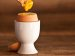Time (Year 4)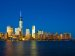Time (Year 5)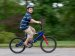Time (Year 6)

### Have Fun with KS2 Maths

Maths does not have to be boring! Watch the video to see how you can learn by playing quizzes.